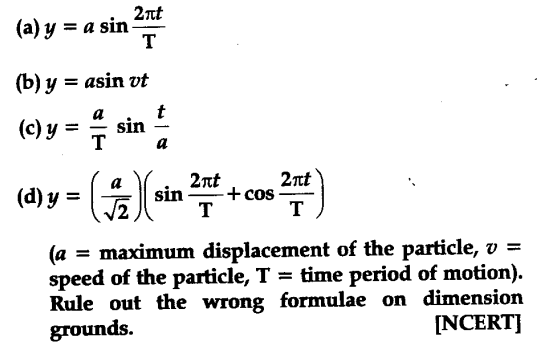# A book with many printing errors contains four different formulae for the displacement y of a particle undergoing a certain periodic motion:

A book with many printing errors contains four different formulae for the displacement y of a particle undergoing a certain periodic motion:According to the principle of homogeneity of dimensions, the dimensions of L.H.S. = dimensions of R.H.S. of equation. Also argument of a trigonometric function should be dimensionless. Thus relation (a) and (d) are true to the above facts whereas (b) and © are false.